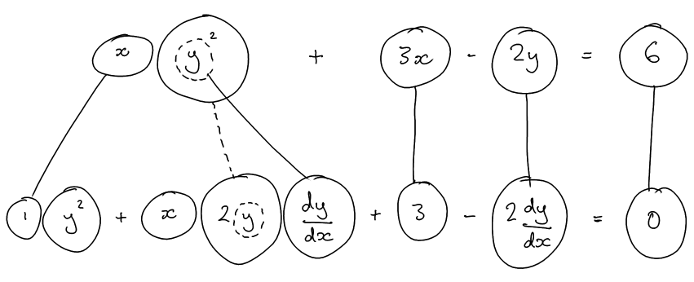# Thread: Find the equation of a tangent

1. ## Find the equation of a tangent

I need to find the equation of the tangent to the curve xy2 + 3x -2y=6. at the point (2,1)

I know I need to differentiate implicitly but its getting confusing differentiating the xy2
After differentiation then id set the function dy/dx to equal 0?

2. ## Re: Find the equation of a tangentOriginally Posted by kmgrosvenorI know I need to differentiate implicitly but its getting confusing differentiating the xy2
Do you know how to use the product rule?

$\displaystyle \frac d{dx}\left[xy^2\right] = x\cdot\frac d{dx}\left[y^2\right] + y^2\cdot\frac{dx}{dx}$

$\displaystyle =2xy\frac{dy}{dx} + y^2$

3. ## Re: Find the equation of a tangent

Just in case a picture (also) helps...... where (key in spoiler) ...

Spoiler:... is the chain rule. Straight continuous lines differentiate downwards (integrate up) with respect to the main variable (in this case x), and the straight dashed line similarly but with respect to the dashed balloon expression (the inner function of the composite which is subject to the chain rule).

But this is wrapped inside the legs-uncrossed version of...... the product rule, where, again, straight continuous lines are differentiating downwards with respect to x.

PS:Originally Posted by kmgrosvenorAfter differentiation then id set the function dy/dx to equal 0?
No, that would be to find a stationary point. (Fooled me for a minute, though!)

Solve the bottom row for dy/dx, then plug in the given value for each of x and y.

__________________________________________________ __________

Don't integrate - balloontegrate!

Balloon Calculus; standard integrals, derivatives and methods

Balloon Calculus Drawing with LaTeX and Asymptote!

4. ## Re: Find the equation of a tangent

Ok so I get the rule.
y^2 + x.2y dy/dx +3 -2 dy/dx =0

Id bring over the 3 so itd be -3
2xy^2 dy/dx + y^2 - 2 dy/dx = -3 is this right?
Grouping them or multiplying them is confusing..

2xy^2-2 dy/dx + y2 = -3 ??

5. ## Re: Find the equation of a tangent

Oh.. i need to take the squared off of the 2xy..

6. ## Re: Find the equation of a tangentOriginally Posted by kmgrosvenorOk so I get the rule.
y^2 + x.2y dy/dx +3 -2 dy/dx =0
Correct.Originally Posted by kmgrosvenorId bring over the 3 so itd be -3
2xy dy/dx + y^2 - 2 dy/dx = -3 is this right?
I.e. "subtract 3 from each side" yes!

(After correcting your typo, which I missed, well spotted.)

Also, subtract y^2 from each side.

Then bring dy/dx outside of a pair of brackets on the left hand side... i.e. factorise.

Then divide both sides by the bracketted expression/sum.

7. ## Re: Find the equation of a tangent

I got it ! Thanks so much

8. ## Re: Find the equation of a tangent

Wait hold on, so i have a fraction with x's and y's.. plug in the (2,1) at the same time?

9. ## Re: Find the equation of a tangentOriginally Posted by kmgrosvenorWait hold on, so i have a fraction with x's and y's.. plug in the (2,1) at the same time?
Yes.

#### Search Tags

calculus, equation, stationary points, tangent